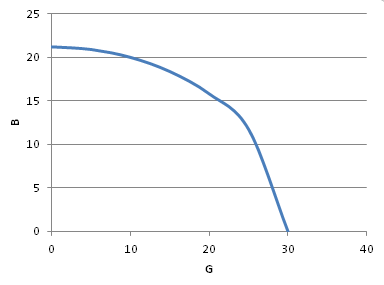# Suppose the production possibility frontier for guns (G) and better (B) is given by G^2 + 2B^2 =...

## Question:

Suppose the production possibility frontier for guns (G) and better (B) is given by

{eq}G^2 + 2B^2 = 900 {/eq}. If you put G on the X axis, and B on Y axis, the slope of the tangent line at each point on PPF is -G/2B

a) Graph the production possibility frontier.

b) If individual's preference is U = G + 2B. How much B and G will be produced? Graph this allocation in the same graph as in part a.

## Production Possibility Frontier:

The production possibility frontier (PPF) describes the set of goods an economy can produce with its endowment of resources and the level of production technology. The slope of the production possibility frontier indicates the marginal rate of technical substitution.

Become a Study.com member to unlock this answer!

a) The possibility frontier is plotted below:b) The optimal production is such that the marginal rate of technical substitution is equal to the...Using the Production Possibility Curve to Illustrate Economic Conditions

from

Chapter 3 / Lesson 8
16K

The production possibility curve demonstrates the potential profit from a given economic condition. See how this illustrates different economic conditions through evaluating scarcity, production factors, efficiency, and opportunity costs.